Question

A buffer that contains 0.27 M of a base, B and 0.44 M of its conjugate acid BH+, has a pH of 9.05. What is the pH after 0.03 mol of NaOH are added to 0.79 L of the solution?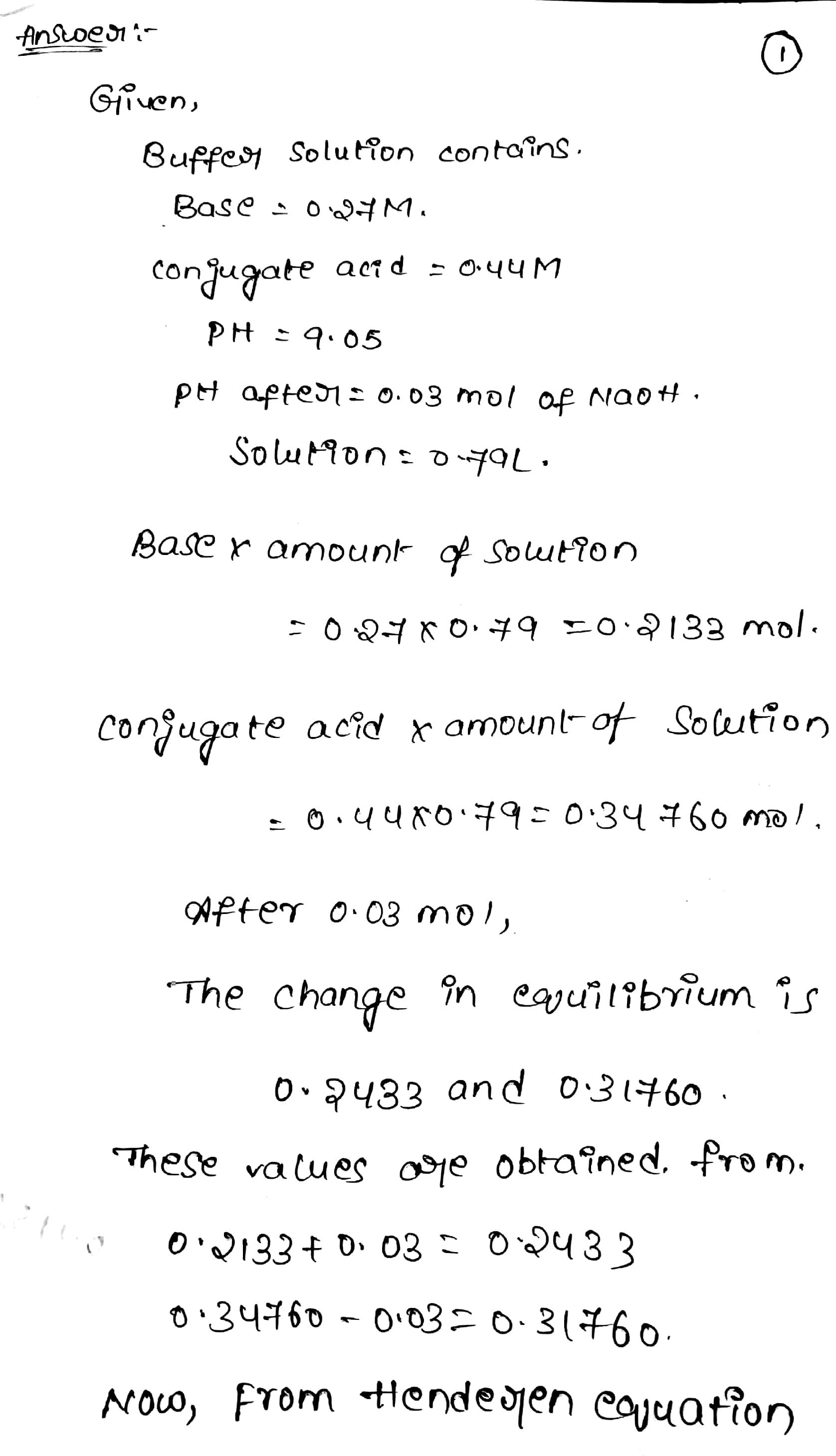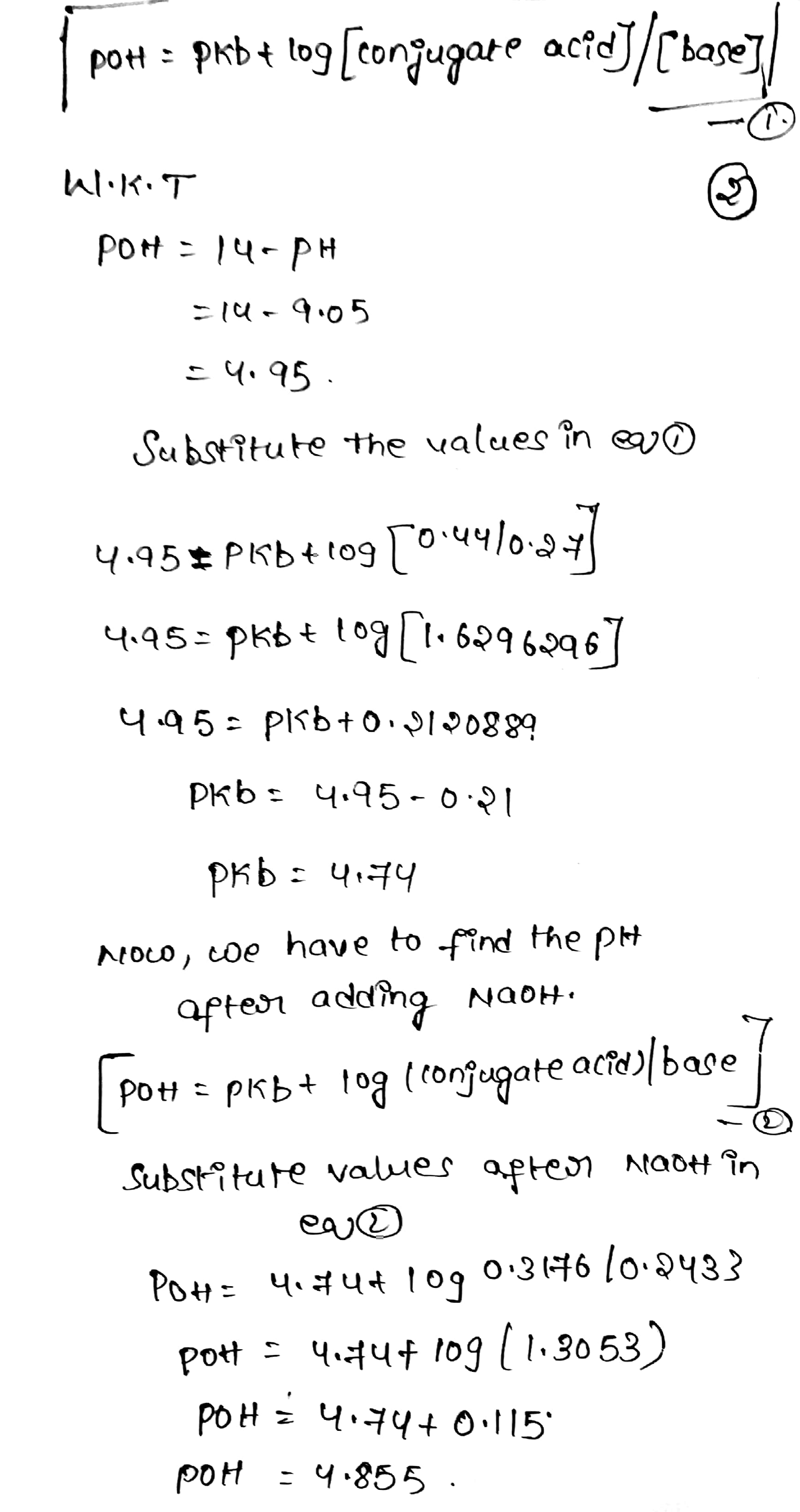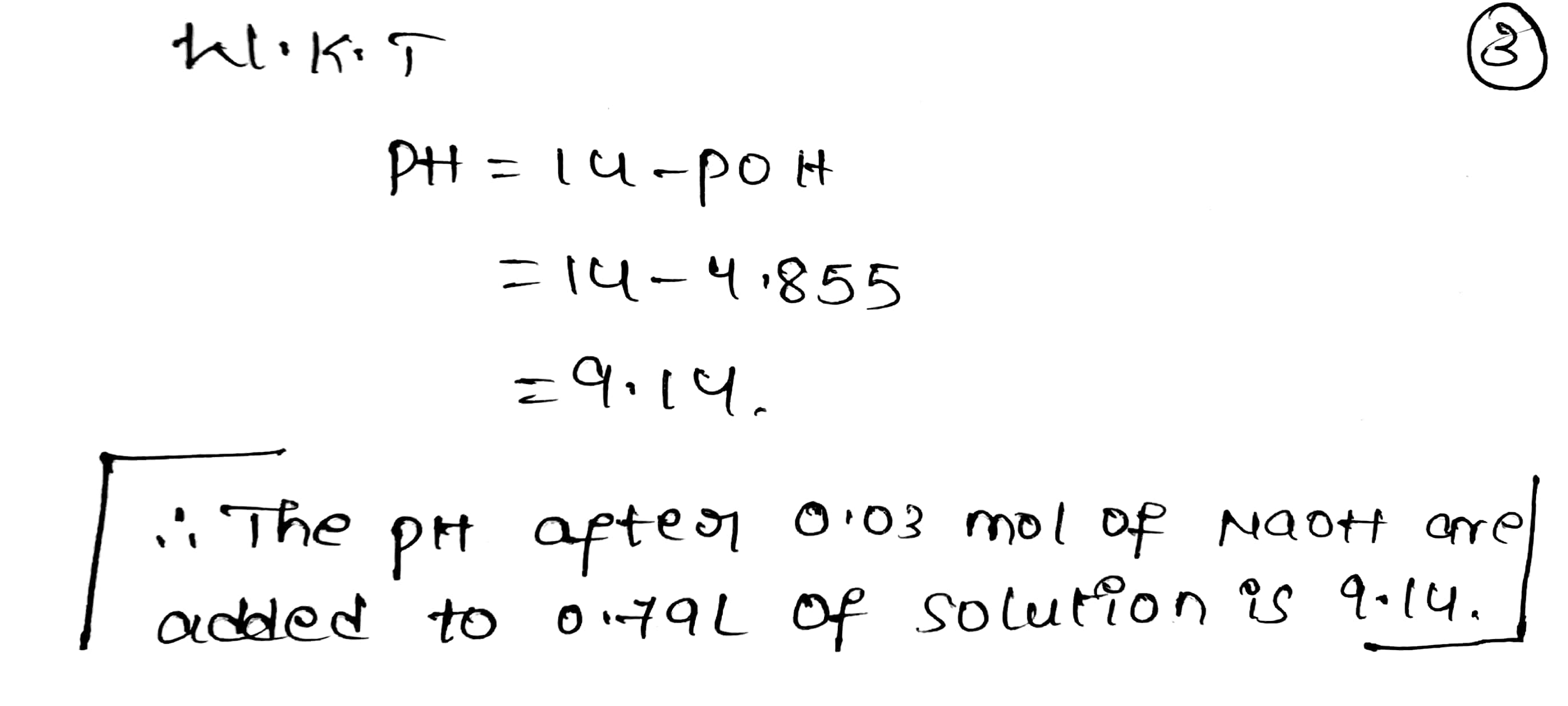#### Earn Coins

Coins can be redeemed for fabulous gifts.

Similar Homework Help Questions
• ### A buffer that contains 0.470 M base, B, and 0.343 M of its conjugate acid, BH,...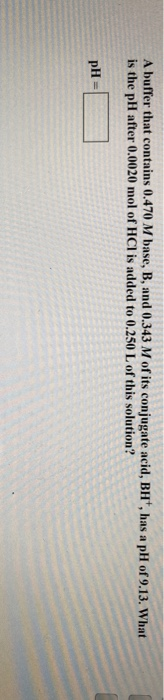A buffer that contains 0.470 M base, B, and 0.343 M of its conjugate acid, BH, has a pH of 9.13. What is the pH after 0.0020 mol of HCl is added to 0.250 L of this solution? pH =

• ### A buffer that contains 1.05 M base, B, and 0.750 M of its conjugate acid, BH+,...

A buffer that contains 1.05 M base, B, and 0.750 M of its conjugate acid, BH+, has a pH of 9.250. What is the pH after 0.0055 mol of HCl is added to 0.400 L of this solution?

• ### A buffer that contains 1.00 M base, B, and 0.750 M of its conjugate acid, BH^+,...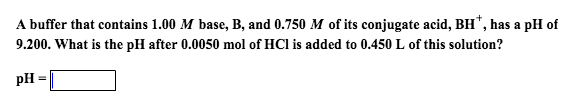A buffer that contains 1.00 M base, B, and 0.750 M of its conjugate acid, BH^+, has a pH of 9.200. What is the pH after 0.0050 mol of HCl is added to 0.450 L of this solution?

• ### A buffer that contains 0.550 M base, B, and 0.229 M of its conjugate acid, BH+, has a pH of 8.90. What is the pH after 0...

A buffer that contains 0.550 M base, B, and 0.229 M of its conjugate acid, BH+, has a pH of 8.90. What is the pH after 0.0020 mol of HCl is added to 0.250 L of this solution?

• ### Enter your answer in the provided box. A buffer that contains 0.388 M base, B, and 0.283 M of its conjugate acid, BH+,...

Enter your answer in the provided box. A buffer that contains 0.388 M base, B, and 0.283 M of its conjugate acid, BH+, has a pH of 8.99. What is the pH after 0.0020 mol of HCl is added to 0.250 L of this solution? pH =

• ### Enter your answer in the provided box. A buffer that contains 0.368 M base, B, and 0.229 M of its conjugate acid, BH...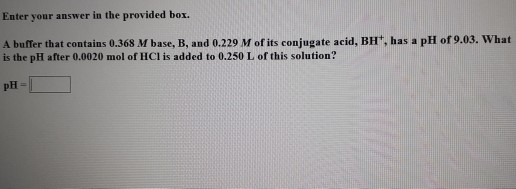Enter your answer in the provided box. A buffer that contains 0.368 M base, B, and 0.229 M of its conjugate acid, BH, has a pH of 9.03. What is the pH after 0.0020 mol of HCl is added to 0.250 L of this solution?

• ### Enter your answer in the provided box. A buffer that contains 1.10 M base, B, and 0.700 M of its conjugate acid, BH, ha...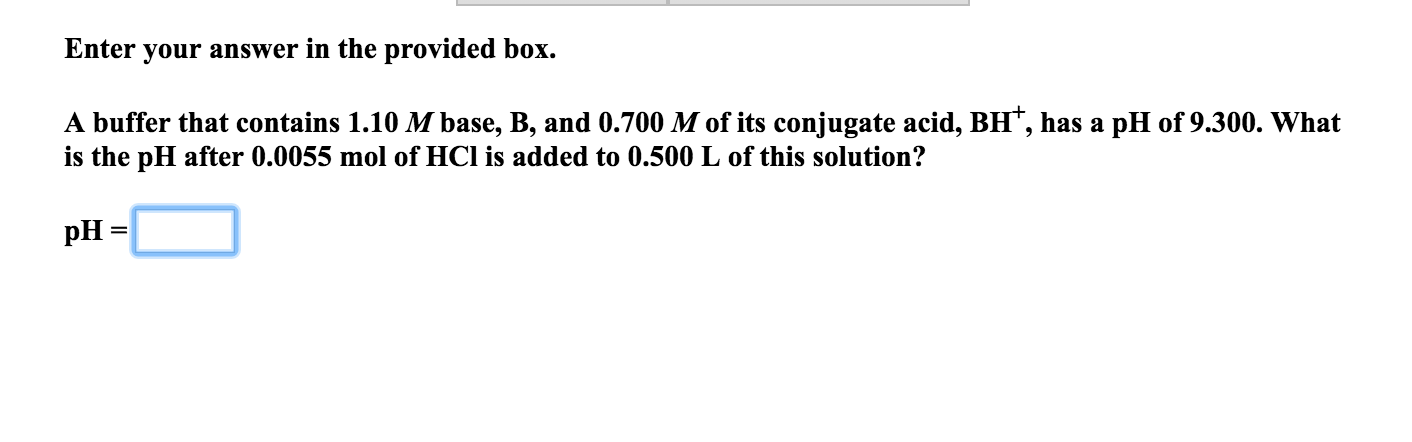Enter your answer in the provided box. A buffer that contains 1.10 M base, B, and 0.700 M of its conjugate acid, BH, has a pH of 9.300. What is the pH after 0.0055 mol of HCl is added to 0.500 L of this solution? pH =

• ### Enter your answer in the provided box. A buffer that contains 0.459 M base, B, and...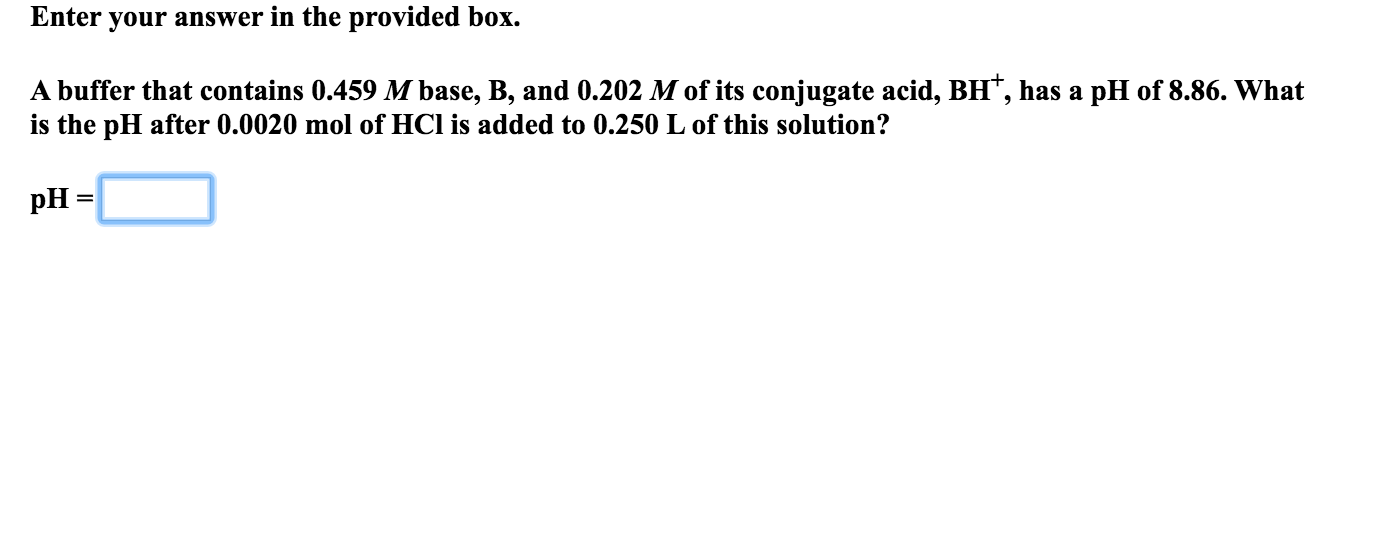Enter your answer in the provided box. A buffer that contains 0.459 M base, B, and 0.202 M of its conjugate acid, BH, has a pH of 8.86. What is the pH after 0.0020 mol of HCl is added to 0.250 L of this solution? pH =

• ### Enter your answer in the provided box. A buffer that contains 1.05 M base, B, and...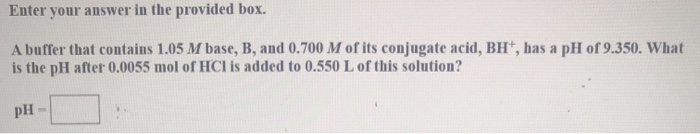Enter your answer in the provided box. A buffer that contains 1.05 M base, B, and 0.700 M of its conjugate acid, BH, has a pH of 9.350. What is the pH after: 0.0055 mol of HCl is added to 0.550 L of this solution? pH

• ### Enter your answer in the provided box. A buffer that contains 0.564 M base, B, and...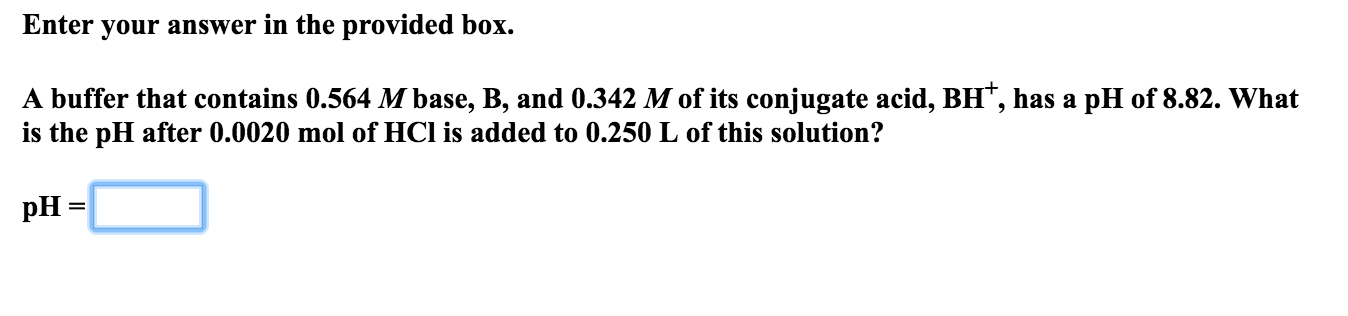Enter your answer in the provided box. A buffer that contains 0.564 M base, B, and 0.342 M of its conjugate acid, BH, has a pH of 8.82. What is the pH after 0.0020 mol of HCl is added to 0.250 L of this solution? pH =